Euler-Lagrange Equation:

Theorem 1: If y (x) is a curve in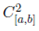which minimize the functional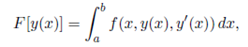Then the following differential equations must be satiesfied: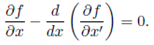The equations is called the Euler Lagrange Equations. Before providing this theorem, we consider an example.

Example1: If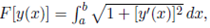Then the Euler Lagrange Equations is given by: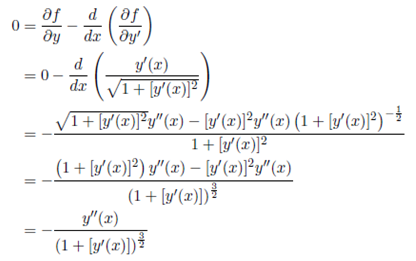The Chain Rule:

We begin with a review of the chain rule for function of one variable. Suppose f (x) is a differential functions of x and x = x (t) is differentiable of t. By the Chain rule theorem the composition functions z (t) = fo x (t) is a differential function of t and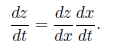For example if f (x) = sin (x) and x (t) = t2, then derivatives with respect to t of z = sin (t2) is given by cos (t2) 2t. It turns out that there is a chain rule for function of several variables. For example suppose x and y are function of t and consider the functions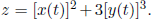We can think of z as the composition of the functions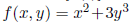with in the functions x (t) and y (t). By a chain rule theorem for function of several variables,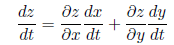Note the similarity function between the above equations. For function of several variables one need to keep track of each of the independent variables seperately applying a chain rule to each. The result for the chain rule theorem for the required functions z = f (x,y) to have continuous partial derivatives and for x (t) and y (t) to be differentiable.